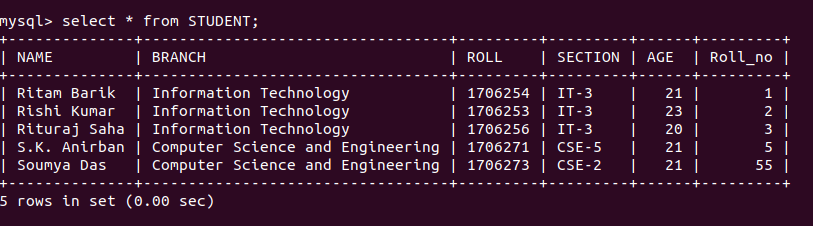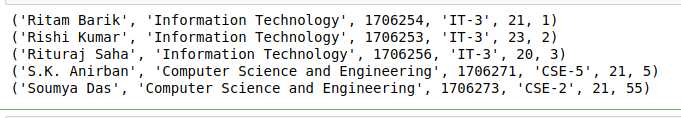# Connect to MySQL using PyMySQL in Python

• Last Updated : 05 Sep, 2020

In this article, we will discuss how to connect to the MySQL database remotely or locally using Python. In below process, we will use PyMySQL module of Python to connect our database.

## What is PyMySQL?

This package contains a pure-Python MySQL client library, based on PEP 249.

Requirements :

MySQL Server – one of the following :

• MySQL >= 5.5
• MariaDB >= 5.5

Installation :

This module does not come built-in with Python. You have to install it externally. To install this type the below command in the terminal.

```pip install PyMySQL
```

## Connecting to MySQL

The proper way to get an instance of this class is to call connect() method. This method establishes a connection to the MySQL database and accepts several arguments:

Parameters :

• host – Host where the database server is located
• database – Database to use, None to not use a particular one.
• port – MySQL port to use, default is usually OK. (default: 3306)

Example 1: Let’s connect to the MySQL server.

## Python3

 `import` `pymysql`` ` `def` `mysqlconnect():``    ``# To connect MySQL database``    ``conn ``=` `pymysql.connect(``        ``host``=``'localhost'``,``        ``user``=``'root'``, ``        ``password ``=` `"pass"``,``        ``db``=``'College'``,``        ``)``     ` `    ``cur ``=` `conn.cursor()``    ``cur.execute(``"select @@version"``)``    ``output ``=` `cur.fetchall()``    ``print``(output)``     ` `    ``# To close the connection``    ``conn.close()`` ` `# Driver Code``if` `__name__ ``=``=` `"__main__"` `:``    ``mysqlconnect()`

Output :

```(('5.7.30-0ubuntu0.18.04.1',),)
```

Example 2: Let’s try to access a table from the database

Table used:## Python3

 `import` `pymysql`` ` `def` `mysqlconnect():``    ``# To connect MySQL database``    ``conn ``=` `pymysql.connect(``        ``host``=``'localhost'``,``        ``user``=``'root'``, ``        ``password ``=` `"pass"``,``        ``db``=``'College'``,``        ``)``     ` `    ``cur ``=` `conn.cursor()``     ` `    ``# Select query``    ``cur.execute(``"select * from STUDENT"``)``    ``output ``=` `cur.fetchall()``     ` `    ``for` `i ``in` `output:``        ``print``(i)``     ` `    ``# To close the connection``    ``conn.close()`` ` `# Driver Code``if` `__name__ ``=``=` `"__main__"` `:``    ``mysqlconnect()`

Output:My Personal Notes arrow_drop_up